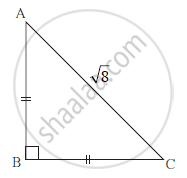Advertisement Remove all ads

# With the Help of the Information Given in the Figure, Fill in the Boxes to Find Ab and Bc . - GeometryWith the help of the information given in the figure, fill in the boxes to find AB and BC .

AB = BC                    (Given)

∴∠ BAC = ∠ BCA =
∴ AB = BC =    × AC
× sqrt8
× 2sqrt2
= 2

Advertisement Remove all ads

#### Solution

AB = BC
∠ BAC = ∠ BCA =45˚

AB = BC = 1/sqrt2 × AC

= 1/sqrt2 × 8 = 1/sqrt2 × sqrt(4×2)

= 1/sqrt2 × 2sqrt2
= 2

Concept: Similar Triangles
Is there an error in this question or solution?
Advertisement Remove all ads
Advertisement Remove all ads
Share
Notifications

View all notifications

Forgot password?